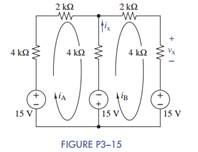### Create an Account

Already have account?

### Forgot Your Password ?

Home / Questions / Formulate mesh-current equations for the circuit in Figure P3 15 Arrange the results in m...

# Formulate mesh-current equations for the circuit in Figure P3 15 Arrange the results in matrix form Ax = b b Solve for iA and iB c Use these results to find

Formulate mesh-current equations for the circuit in Figure P3−15. Arrange the results in matrix form Ax = b.

(b) Solve for iA and iB.

(c) Use these results to find vx and ix.Jun 15 2020 View more View Less

#### Answer (Solved)Subscribe To Get Solution